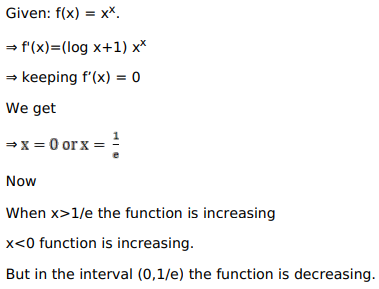# Solve this following

Question:

Mark $(\sqrt{)}$ against the correct answer in the following:

$f(\mathrm{x})=\mathrm{x}^{\mathrm{x}}$ is decreasing in the interval

A. $(0, \mathrm{e})$

B. $\left(0, \frac{1}{\mathrm{e}}\right)$

C. $(0,1)$

D. none of these

Solution: